Select Page

# Maths Continuity and Differentiability CBSE 12 Science MCQ in English Answers

Maths Continuity and Differentiability CBSE 12 Science MCQ in English Answers to enable students to get Answers in a narrative video format for the specific question.

Expert Teacher provides Maths Continuity and Differentiability CBSE 12 Science MCQ English Answers. This video solution will be useful for students to understand how to write an answer in exam in order to score more marks. This teacher uses a narrative style for a question from Continuity and Differentiability not only to explain the proper method of answering question, but deriving right answer too.

Please find the question below and view the Answer in a narrative video format.

Question:

## Similar Questions from CBSE, 12th Science, Maths, Continuity and Differentiability

Question 1 : Differentiate w.r.t.x the function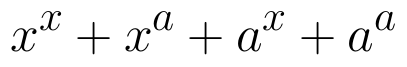, for some fixed a > 0 and x > 0. (View Answer Video)

Question 2 : Differentiate the function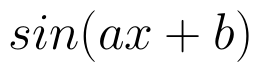with respect to x. (View Answer Video)

Question 3 : If x and y are connected parametrically by the equation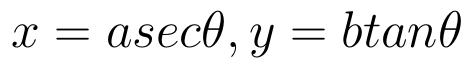, without eliminating the parameter, find. (View Answer Video)

Question 4 : If x and y are connected parametrically by the equation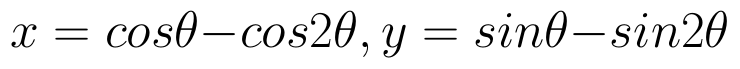, without eliminating the parameter, find. (View Answer Video)

Question 5 : Differentiate the function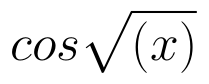with respect to x. (View Answer Video)

### Determinant

Question 1 : The solution of the following system of equation is 2x + 3y = 5, 5x – 2y = 3. (View Answer Video)

Question 2 : Let A be a non-singular square matrix of order 3 * 3. Then | adj A | is equal to: (View Answer Video)

Question 3 : Evaluate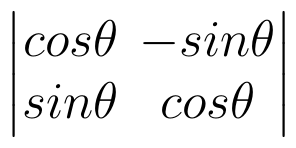. (View Answer Video)

Question 4 : Evaluate the determinant: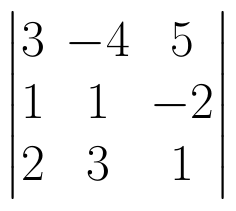. (View Answer Video)

### Differential Equations

Question 2 : Find the particular solution of the differential equation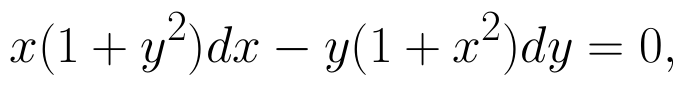given that y = 1 when x = 0.  (View Answer Video)

Question 3 : Determine degree of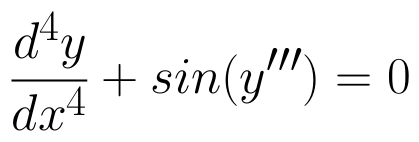(View Answer Video)

Question 4 : Find the particular solution of the differential equation :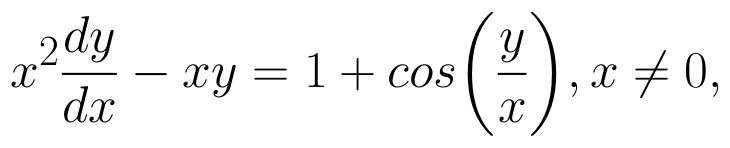when x = 1,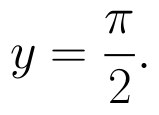(View Answer Video)

Question 5 : Write the differential equation representing the curve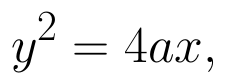where a is an arbitrary constant. (View Answer Video)

### Integrals

Question 1 : Find the integral of the function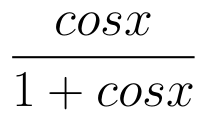. (View Answer Video)

Question 2 : Evaluate :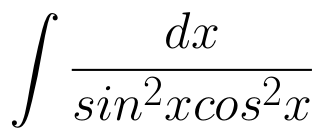. (View Answer Video)

Question 3 : Find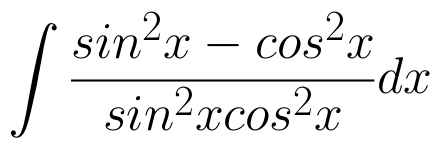. (View Answer Video)

Question 4 : Evaluate :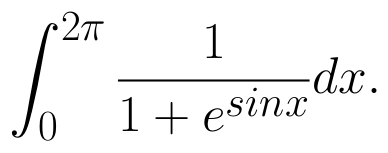(View Answer Video)

Question 5 : Find the integral of the function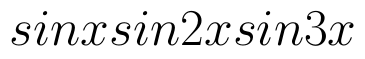. (View Answer Video)